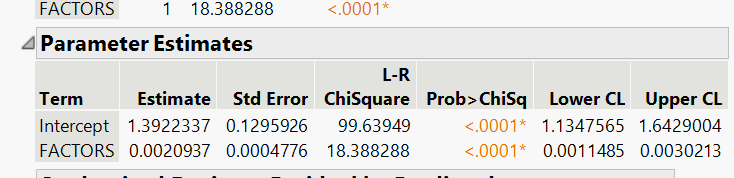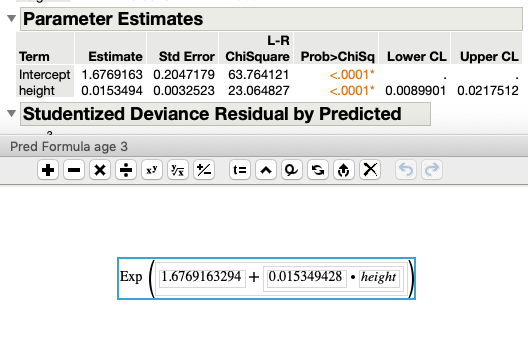Choose Language Hide Translation Bar
Highlighted

## Poisson's Regression equation (e^B0+B1x1????)with Generalized Linear Model

Hi can someone give me a hand?

So we are doing Poisson's Regression right now- we were asked to use the Generalized Linear Model - Distribution: Poisson's - Link function: log. My teacher has asked to write the regression equation with no guidance on how to do so. His advice was go do it in SAS, which would be useful if 1). I had a SAS license and 2). knew how to code in SAS. But without those I am left to JMP, which is in fact what the class is about so hopefully my frustration is understood.

I understand its theoretically "linear" and I understand log is used for our link but can someone help me write it out. I am finding conflicting errors online and I can't complete any of my homework with out this.

My y variable is Length of Stay in hospital, my X-variable is number of factors (number of medical items used during stay)  My parameters are as followed:I attempted to use the "prediction formula column to give me the formula, which said e^B0+B1x1 --> e^1.392+0.0021x. Is this what it is?

Also if whoever answers this has a solid understanding on Poisson's, I have an additional question on model adequacy.

THANK YOU

#STATISTICS

Highlighted

## Re: Poisson's Regression equation (e^B0+B1x1????)with Generalized Linear Model

Parameter Estimates and the Saved Prediction FormulaThis is in JMP v15.  Maybe in earlier versions of JMP the formula was expressed differently.

Since the link function is Log, the prediction must be exponentiated back to units of X.

Not a Poisson expert, but its a fun distribution because the center and scale parameters are equal. So when X is big it looks normal, and when X is close to 0 it looks log normal.

This is from the scripting index, super useful for understanding the behavior of the distribution at different levels of lambda

``````Names Default To Here( 1 );
lambda = 4;
New Window( "Example: Poisson Probability",
pdy = Graph Box(
Y Scale( 0, 0.20 ),
X Scale( -1, 40 ),
Pen Color( "red" ),
Pen Size( 2 );
For( k = 0, k <= 40, k++,
V Line(
k,
0,
Poisson Probability( lambda, k )
)
);
Text(
{30, 0.18},
"\!U03BB=",
Round( lambda, 2 )
);
),
H List Box(
Slider Box( 0, 40, lambda, pdy << reshow ),
Text Box( " \!U03BB" )
)
);``````
JMP Systems Engineer, Pharm and BioPharm Sciences
Article Labels